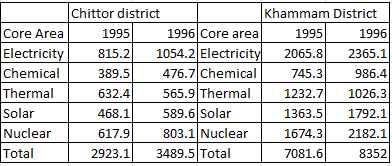### CAT 1996 Question Paper Question 64

Instructions

Answer the questions based on the following information. The amount of money invested (rupees in crores) in the core infrastructure areas of two districts, Chittoor and Khammam, Andhra Pradesh, is as follows.Question 64

# Approximately how many times was the total investment in Chittoor to the total investment in Khammam?

Solution

Total investment in chittoor = 2923.1 + 3489.5 = 6412.6
Total investment in khammam = 7081.6 + 8352 = 15433.6
Ratio = $$15433.6 \div 6412.6 = 2.4$$ (nearly)

• All Quant CAT Formulas and shortcuts PDF
• 30+ CAT previous papers with solutions PDF